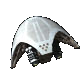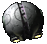## need help with helper functions ?

Freeform discussion about anything related to modding Transcendence.
digdugPosts: 2615
Joined: Mon Oct 29, 2007 9:23 pm
Location: Decoding hieroglyphics on Tan-Ru-Dorem
hello!

How do I write a function that rounds number to the nearest integer ?

digdugPosts: 2615
Joined: Mon Oct 29, 2007 9:23 pm
Location: Decoding hieroglyphics on Tan-Ru-Dorem
This works:

Code: Select all

``````(setq we_round (lambda (input)
(block (tempNumber output)
(setq tempNumber (- input (int input)))
(if (geq tempNumber 0.5)
(setq output (+ 1 (int input)))
(setq output (int input))
)
)))``````
is it efficient ? is there a better way ?

AssumedPseudonym
Fleet OfficerPosts: 1159
Joined: Thu Aug 29, 2013 5:18 am
Location: On the other side of the screen.
That looks to be about the best you’re going to get.Mod prefixes: 0xA010 (registered) and 0xDCC8 (miscellaneous)

Of all the things I’ve lost in life, I miss my mind the least. (I’m having a lot more fun without it!)

giantcabbage
Militia LieutenantPosts: 104
Joined: Thu Apr 07, 2011 9:05 pm
That does not work for negative numbers as int truncates towards zero. Try:

Code: Select all

``````(setq nint (lambda (x) (int (+ x (if (ls x 0) -.5 .5)))))
``````

schilcote
Militia CaptainPosts: 726
Joined: Sat Feb 02, 2008 7:22 pm
My lisp is incredibly rusty, so this might have errors, but here's a better way:

Code: Select all

``````(setq schilround (lambda (x)
(block (modresult roundresult)
(setq modresult (modulo x 1) ;Change this 1 to any other power of 10 to round to that, i.e. 0.1 rounds to 1 decimal place
(setq roundresult (sub x modresult))
(if geq (modresult 0.5) ;If you change the above number you should also change this number similarly
(setq roundresult (add roundresult 1) ;As well as this one
)
))
``````
For example, 12.89 modulo 1 = 0.89.
Therefore, 12.89 - 12.89 modulo 1 = 12. That gives you a floor function.
Then you check if the decimal part was greater than 0.5 to know if you should round up. I'm sure there's a smarter way to do that but I'm not clever enough.

Oh, and as the comments suggest, scaling all those constants will allow you to change where the function round to, i.e. if I change 1 to 0.1 and 0.5 to 0.05, it'll round to one decimal place instead of 0. Conversely, 1 to 10 and 0.5 to 5 will round to the nearest ten (i.e. 123 becomes 120).
[schilcote] It doesn't have to be good, it just has to not be "wow is that the only thing you could think of" bad

digdug``````(setq nint (lambda (x) (int (+ x (if (ls x 0) -.5 .5)))))
thanks !!